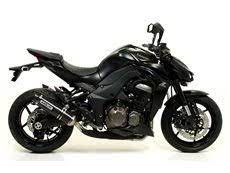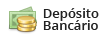# Ponteira dupla Arrow Thunder Steel end cap - Kawasaki Z 1000 17'~21'

Código: 71755 Marca:
Selecione a opção de Ponteira :
6x de R\$ 1.353,33
ou R\$ 7.470,40 via Depósito
Comprar Estoque: 60 dias úteis
6x de R\$ 1.048,33
ou R\$ 5.786,80 via Depósito
Comprar Estoque: 60 dias úteis
6x de R\$ 1.048,33
ou R\$ 5.786,80 via Depósito
Comprar Estoque: 60 dias úteis
6x de R\$ 1.353,33
ou R\$ 7.470,40 via Depósito
Comprar Estoque: 60 dias úteis
6x de R\$ 1.048,33
A partir de R\$ 6.290,00
ou R\$ 5.786,80 via Depósito
• 1x de R\$ 8.120,00 sem juros
• 2x de R\$ 4.060,00 sem juros
• 3x de R\$ 2.706,66 sem juros
• 4x de R\$ 2.030,00 sem juros
• 5x de R\$ 1.624,00 sem juros
• 6x de R\$ 1.353,33 sem juros
• 1x de R\$ 8.120,00 sem juros
• 2x de R\$ 4.060,00 sem juros
• 3x de R\$ 2.706,66 sem juros
• 4x de R\$ 2.183,97
• 5x de R\$ 1.772,53
• 6x de R\$ 1.498,43
• 7x de R\$ 1.302,82
• 8x de R\$ 1.156,25
• 9x de R\$ 1.042,39
• 10x de R\$ 951,42
• 11x de R\$ 877,10
• 12x de R\$ 815,26
• R\$ 7.470,40• 1x de R\$ 6.290,00 sem juros
• 2x de R\$ 3.145,00 sem juros
• 3x de R\$ 2.096,66 sem juros
• 4x de R\$ 1.572,50 sem juros
• 5x de R\$ 1.258,00 sem juros
• 6x de R\$ 1.048,33 sem juros
• 1x de R\$ 6.290,00 sem juros
• 2x de R\$ 3.145,00 sem juros
• 3x de R\$ 2.096,66 sem juros
• 4x de R\$ 1.691,77
• 5x de R\$ 1.373,05
• 6x de R\$ 1.160,73
• 7x de R\$ 1.009,20
• 8x de R\$ 895,67
• 9x de R\$ 807,47
• 10x de R\$ 737,00
• 11x de R\$ 679,43
• 12x de R\$ 631,52
• R\$ 5.786,80• 1x de R\$ 6.290,00 sem juros
• 2x de R\$ 3.145,00 sem juros
• 3x de R\$ 2.096,66 sem juros
• 4x de R\$ 1.572,50 sem juros
• 5x de R\$ 1.258,00 sem juros
• 6x de R\$ 1.048,33 sem juros
• 1x de R\$ 6.290,00 sem juros
• 2x de R\$ 3.145,00 sem juros
• 3x de R\$ 2.096,66 sem juros
• 4x de R\$ 1.691,77
• 5x de R\$ 1.373,05
• 6x de R\$ 1.160,73
• 7x de R\$ 1.009,20
• 8x de R\$ 895,67
• 9x de R\$ 807,47
• 10x de R\$ 737,00
• 11x de R\$ 679,43
• 12x de R\$ 631,52
• R\$ 5.786,80• 1x de R\$ 8.120,00 sem juros
• 2x de R\$ 4.060,00 sem juros
• 3x de R\$ 2.706,66 sem juros
• 4x de R\$ 2.030,00 sem juros
• 5x de R\$ 1.624,00 sem juros
• 6x de R\$ 1.353,33 sem juros
• 1x de R\$ 8.120,00 sem juros
• 2x de R\$ 4.060,00 sem juros
• 3x de R\$ 2.706,66 sem juros
• 4x de R\$ 2.183,97
• 5x de R\$ 1.772,53
• 6x de R\$ 1.498,43
• 7x de R\$ 1.302,82
• 8x de R\$ 1.156,25
• 9x de R\$ 1.042,39
• 10x de R\$ 951,42
• 11x de R\$ 877,10
• 12x de R\$ 815,26
• R\$ 7.470,40• 1x de R\$ 6.290,00 sem juros
• 2x de R\$ 3.145,00 sem juros
• 3x de R\$ 2.096,66 sem juros
• 4x de R\$ 1.572,50 sem juros
• 5x de R\$ 1.258,00 sem juros
• 6x de R\$ 1.048,33 sem juros
• 1x de R\$ 6.290,00 sem juros
• 2x de R\$ 3.145,00 sem juros
• 3x de R\$ 2.096,66 sem juros
• 4x de R\$ 1.691,77
• 5x de R\$ 1.373,05
• 6x de R\$ 1.160,73
• 7x de R\$ 1.009,20
• 8x de R\$ 895,67
• 9x de R\$ 807,47
• 10x de R\$ 737,00
• 11x de R\$ 679,43
• 12x de R\$ 631,52
• R\$ 5.786,80* Este prazo de entrega está considerando a disponibilidade do produto + prazo de entrega.

Ponteira dupla Arrow Thunder Steel end cap - Kawasaki Z 1000 17'~21'